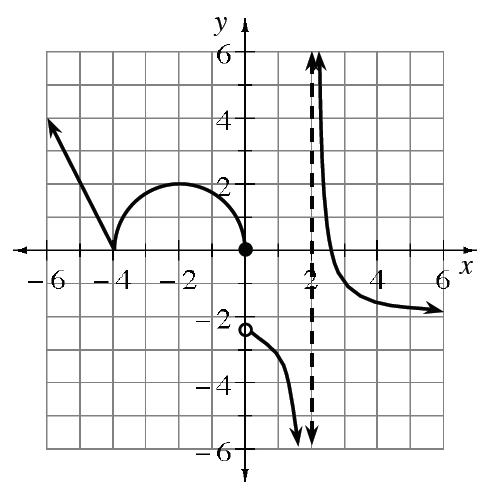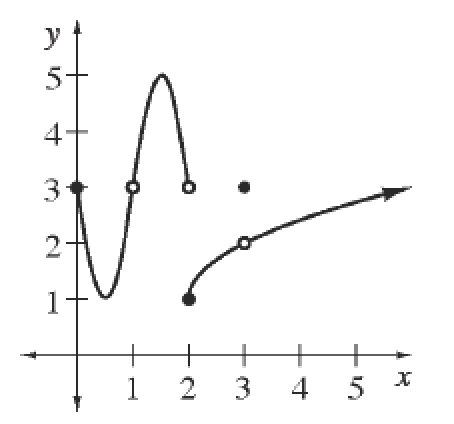### Home > APCALC > Chapter 3 > Lesson 3.1.1 > Problem3-14

3-14.

For the graph in problem 3-13, state the domain and range using interval notation.There are two accepted forms of notation for domain and range. Examine the graph at right. The domain and range of the function shown can be noted in either interval notation or in set notation.

Interval Notation: $D = [0, 1) \cup (1, ∞)$
$R = [1, ∞)$

Set Notation: $D = {x: x ≥ 0 \text{ and } x ≠ 1}$ or $D = {x: 0 ≤ x < 1 \text{ or } x > 1}$
$R = {y: y ≥ 1}$